# BaTiO3晶体薄膜波导极化导模与晶体双折射和波导结构的相关性Correlation of the Polarized Guided Modes of BaTiO3 Crystal Film Waveguide with the Crystal Birefringence and Waveguide Structure

DOI: 10.12677/APP.2021.111008, PDF, HTML, XML, 下载: 78  浏览: 473

Abstract: Barium titanate (BaTiO3), as a ferroelectric crystal with high electro-optical effect, has become one of the frontiers and hot topics in the field of optoelectronic devices in recent years. This paper reports on an investigation of the correlations of polarization modes with the birefringence and structure of BaTiO3 crystal film waveguides. In theory, the relation between the optical polarized mode and the birefringence of BaTiO3 crystal film waveguide structure is modeled, and then the numerical simulations are carried out. In experiments, the different polarization modes of two different waveguide structures are observed. Further, based on the theoretical and experimental results, the correlations of the polarized modes with both the birefringence and waveguide structure are analyzed.

1. 引言

2. 理论原理

BaTiO3晶体薄膜生长技术主要有激光脉冲沉积(Pulsed Laser Deposition, PLD)和金属–有机物–化学气相沉积(Metal-Organic Chemical Vapor Deposition, MOCVD)两种。我们的波导结构是使用PLD技术在0.5 mm厚的氧化镁(MgO)晶体衬底上生长了450 nm厚的BaTiO3薄膜，并在薄膜上直接刻蚀出脊型波导结构。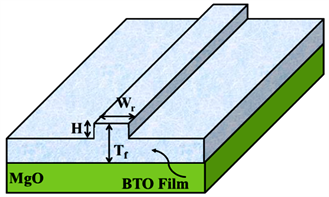Figure 1. Schematic straight rib waveguide structure of BaTiO3 crystal film

BaTiO3晶体与其它双折射晶体相同，其水平偏振态和垂直偏振态的折射率( ${n}_{x}$${n}_{y}$ )之差形成了光束在传输中的双折射值，定义为 $\delta {n}_{xy}={n}_{y}-{n}_{x}$。在如图2所示的x-y坐标系中，由该双折值引起了光波的水平偏振态和垂直偏振态的合成光波偏振态在传输过程中具有不同的光相位值， $\Delta {\varphi }_{xy}=2\text{π}\delta {n}_{xy}/\lambda$ ( $\lambda$ 为光波长)，进而引起了如图2所示的合成光波电场矢量的椭圆分布，其中在原坐标系统的长短轴的半长度分别为 ${A}_{x}$${A}_{y}$，与两个方向偏振态相位差 $\Delta {\varphi }_{xy}$ 共同决定椭圆方位角 ${\alpha }_{ellps}$，由坐标X与Y旋转后的长短轴半长度ab决定椭圆的形状，图中 ${\epsilon }_{ellps}$ 代表旋转的坐标系统中ab形成的椭圆方位角。于是，椭圆方位角 ${\alpha }_{ellps}$ 由下面的方程定义 ：

$\mathrm{tan}2{\alpha }_{ellps}=\frac{2{A}_{x}{A}_{y}}{{A}_{x}^{2}-{A}_{y}^{2}}\mathrm{cos}\Delta {\varphi }_{xy}$ (1)

$\frac{{X}^{2}}{{a}^{2}}+\frac{{Y}^{2}}{{b}^{2}}=1$ (2)

${a}^{2}={A}_{x}^{2}{\mathrm{cos}}^{2}{\alpha }_{ellps}+{A}_{y}^{2}{\mathrm{sin}}^{2}{\alpha }_{ellps}+{A}_{x}{A}_{y}\mathrm{sin}2{\alpha }_{ellps}\mathrm{cos}{\varphi }_{xy}$ (3)

${b}^{2}={A}_{x}^{2}{\mathrm{sin}}^{2}{\alpha }_{ellps}+{A}_{y}^{2}{\mathrm{cos}}^{2}{\alpha }_{ellps}-{A}_{x}{A}_{y}\mathrm{sin}2{\alpha }_{ellps}\mathrm{cos}{\varphi }_{xy}$ (4)

$ab=±{A}_{x}{A}_{y}\mathrm{sin}{\varphi }_{xy}$ (5)Figure 2. Schematic ellipse of the electric field polarization of light-wave in a briefringent crystal

3. 实验与分析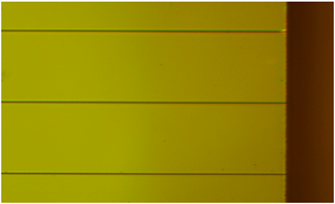Figure 3. Image of the waveguide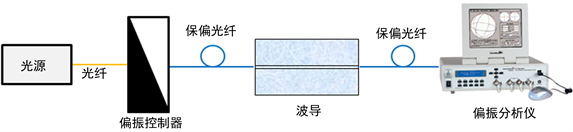Figure 4. Schematic of experimental device(a)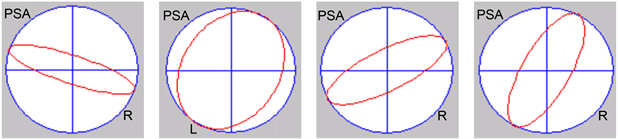(b)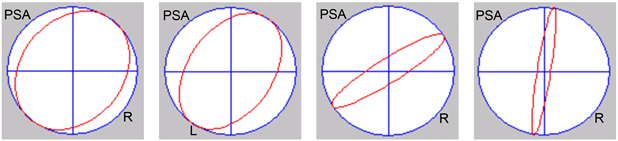(c)

Figure 5. The experimental results of optical polarization are obtained with different ridge widths. (a) Four different polarization states of input light; (b) The output polarization of 3 μm waveguide ridge width; (c) The output polarization of 4 μm waveguide ridge width

4. 结论

NOTES

*通讯作者。

  Hwang, S.J., Yu, H.H. and Wang, J. (2004) Electro-Optic Modulators Formed by In-Plane Electric-Field-Poled Polymer Waveguides. Optics Communications, 233, 341-352. https://doi.org/10.1016/j.optcom.2004.01.059  Ma, H., et al. (2002) Polymer-Based Optical Waveguides: Materials, Processing, and Devices. Advanced Material, 14, 1339-1365. https://doi.org/10.1002/1521-4095(20021002)14:19%3C1339::AID-ADMA1339%3E3.0.CO;2-O  Stewart, G. and Laybourn, P. (1978) Fabrication of Ion-Exchanged Optical Waveguides from Dilute Silver Nitrate Melts. IEEE Journal of Quantum Electronics, 14, 930-934. https://doi.org/10.1109/JQE.1978.1069721  陈益新. 集成光学: 理论和技术[M]. 上海: 上海交通大学出版社, 1985.  陈福深. 集成光学器件导论[M]. 北京: 机械工业出版社, 2010.  蔡红星, 雷建国, 朱万彬. 光波导原理与器件[M]. 北京: 清华大学出版社, 2012: 56-63.  魏贤华, 黄文, 接文静, 等. BaTiO3铁电薄膜在硅基片上的取向生长[J]. 硅酸盐学报, 2007, 35(5): 583-587.  张静, 付秀华, 杨飞, 等. BaTiO3晶体薄膜PLD法生长工艺参量研究[J]. 光子学报, 2014, 43(5): 71-75.  蔡玉平, 冯蒙丽, 宋春荣. BaTiO3对称性与电光性能的研究[J]. 军械工程学院学报, 2015(6): 72-75.  Zhang, J., Fu, X.H., Guo, L.J., et al. (2014) Design and Fabrication of BaTiO3 Crystal Thin-Film Waveguides. Key Engineering Mate-rials, 609-610, 201-207. https://doi.org/10.4028/www.scientific.net/KEM.609-610.201  Sun, D.G., Fu, X., Hall T.J., et al. (2013) Analysis for the Nonlinear Electro-Optic Modulation Effect of BaTiO3 Crystal Thin-Film Wave-guide Modulators. Journal of Applied Physics, 113, 5941. https://doi.org/10.1063/1.4803707  Abel, S., Stöferle, T., Marchiori, C., et al. (2013) A Strong Electro-Optically Active Lead-Free Ferroelectric Integrated on Silicon. Nature Communications, 4, 1671. https://doi.org/10.1038/ncomms2695  Huard, S. (1997) Polarized Optical Waves. In: Huard, S., Ed., Polarization of Light, Chapter 1, Wiley Press, New York, 1-35.